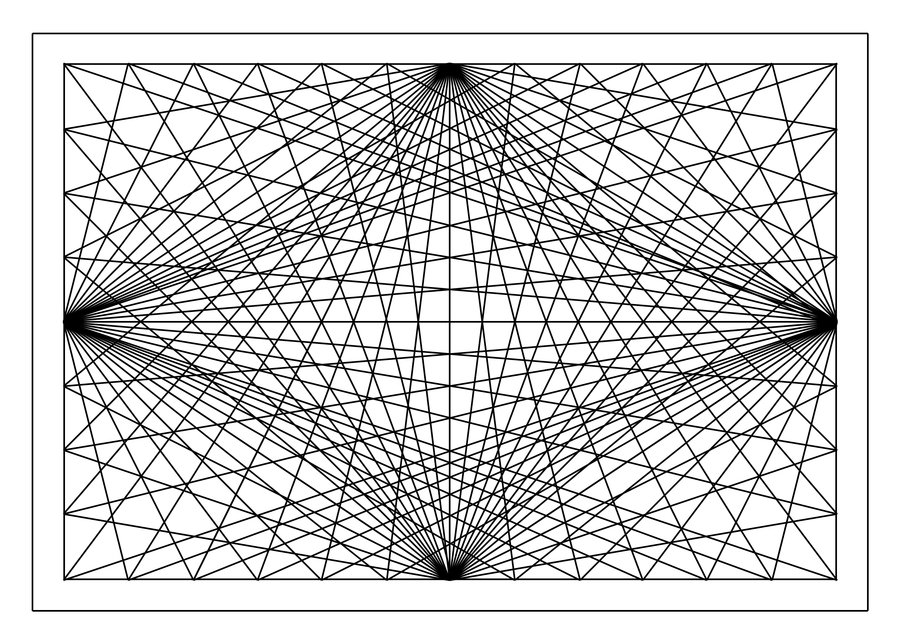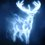# A neat generalization .I found a question which is indeed beautiful in its simplicity. Draw 4 straight lines at random .

a) In how many regions do the lines intersect ?

b) How many triangles are formed ?

c) Into how many regions is the plane divided?Note by Raven Herd
6 years, 4 months ago

This discussion board is a place to discuss our Daily Challenges and the math and science related to those challenges. Explanations are more than just a solution — they should explain the steps and thinking strategies that you used to obtain the solution. Comments should further the discussion of math and science.

When posting on Brilliant:

• Use the emojis to react to an explanation, whether you're congratulating a job well done , or just really confused .
• Ask specific questions about the challenge or the steps in somebody's explanation. Well-posed questions can add a lot to the discussion, but posting "I don't understand!" doesn't help anyone.
• Try to contribute something new to the discussion, whether it is an extension, generalization or other idea related to the challenge.

MarkdownAppears as
*italics* or _italics_ italics
**bold** or __bold__ bold
- bulleted- list
• bulleted
• list
1. numbered2. list
1. numbered
2. list
Note: you must add a full line of space before and after lists for them to show up correctly
paragraph 1paragraph 2

paragraph 1

paragraph 2

[example link](https://brilliant.org)example link
> This is a quote
This is a quote
    # I indented these lines
# 4 spaces, and now they show
# up as a code block.

print "hello world"
# I indented these lines
# 4 spaces, and now they show
# up as a code block.

print "hello world"
MathAppears as
Remember to wrap math in $$ ... $$ or $ ... $ to ensure proper formatting.
2 \times 3 $2 \times 3$
2^{34} $2^{34}$
a_{i-1} $a_{i-1}$
\frac{2}{3} $\frac{2}{3}$
\sqrt{2} $\sqrt{2}$
\sum_{i=1}^3 $\sum_{i=1}^3$
\sin \theta $\sin \theta$
\boxed{123} $\boxed{123}$

Sort by:

Yep, indeed there exists a generalization for it.

Suppose you have $n$ lines in a plane out of which $m$ are parallel (assuming they are not concurrent lines). Then,

a) (I assume you are asking for number of points in which these lines intersect)

So, the number of points in which these lines intersect are ${n \choose 2} - {m \choose 2}$. This is because out of $n$ lines in the plane you can always pick up two of these lines and it's obvious that these lines will intersect at a unique point iff they are not parallel. Thus total number of intersection points will be ${n \choose 2}$ But, notice that we have also counted extra cases because we have some lines parallel. These extra intersection points will be ${m \choose 2}$ So we have to subtract this and thus we get our result.

b) We can always draw a triangle from 3 given points (provided that they are not collinear). And also we know that we will get 3 points from intersection of 3 non-parallel lines. Thus, total number of triangles will be ${n \choose 3}$ . But again we have counted some extra cases because we also have $m$ parallel lines which won't result in 3 intersection points and thus no triangle . Number of these extra triangles will be ${m \choose 3}$. Now also notice that we need to subtract even those cases in which we have only two of the lines parallel. Thus, we will have a total of ${n \choose 3}-{m \choose 3}-{m \choose 2}$ triangles from the intersection of these $n$ lines out of which $m$ are parallel.

c) I'm right now thinking over this case.

Even more generalized case exists when you take $n$ lines in a plane out of which $m_1$ are parallel to each other, then another set $m_2$ lines are parallel $\ldots$ another set of $m_i$ lines are parallel and they are also concurrent. You can even cross-check the above results for small number of cases. I hope, now you'll be able to solve those problems for $n=4, m=0$ case. (I'll soon be coming up with rest of the cases and more generalized results too)

Thanks,

Kishlaya Jaiswal..

- 6 years, 4 months ago

I don't think that is the general case. You've forgotten to account for the fact if the lines are concurrent or if there are more pairs of parallel lines (For example, if the lines make a parallelogram).

Also, in part (b), you forgotten to subtract the case when there are $2$ parallel lines and one line not parallel to them.

- 6 years, 4 months ago

Oh, yes there I see! I have indeed missed many cases. I'll make the edits right now. Thanks for pointing them out. $\ddot \smile$

- 6 years, 4 months ago

Thank you for your work but I need to dwell more on the minute threads of logic which you have implanted in there.Honestly speaking it took me around 1/2 hour to read this and still I constructed an example to understand the little part I fathomed.

- 6 years, 4 months ago